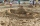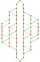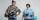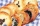# Examples for 9th grade - page 105

1. Sand castleTim and Tom built a sand castle and embellished it with a flag. Half the pole with the flag plunged into the castle. The highest point of the pole was 80 cm above the ground, its lowest point 20 cm above the ground. How high was the sand castle?
2. 15 teachers15 teachers teach for a combined amount of 128 days over a period of 64 days. What is this expressed as a percentage?
3. AlgebraX+y=5, find xy (find the product of x and y if x+y = 5)
4. Price inflationThis year the average wage grew by 4.5%. Prices rose by 2%. How much did the real wage increase in the given year?
5. Mba studiumAt MBA school, fourth-year students can choose from three optional subjects: a) mathematical methods, b) social interaction, c) management Each student studies one of these subjects. The mathematical methods studied 28 students, the social interaction 27.
6. LegsCancer has 5 pairs of legs. The insect has 6 legs. 60 animals have a total of 500 legs. How much more are cancers than insects?
7. DivideDivide area of rectangles with dimensions 32m and 10m by the ratio 7: 9. What area corresponds to a smaller section?
8. Diofant equation250x + 120y = 5640
9. A triangleA triangle has an angle that is 63.1 other 2 are in ratio of 2:5 What are the measurements of the two angles?
10. Midpoint 4If the midpoint of a segment is (6,3) and the other end point is (8,-4) what are thw coordinate of the other end?
11. Two unknown numbersOne number is 2 less than a second number. Twice the second number is 14 less than 5 times the first. Find the two numbers.
12. BlocksThere are 9 interactive basic building blocks of an organization. How many two-blocks combinations are there?
13. Combinations 7A multi-course meal has 3 choices. 3 appetizers, 4 main courses, and 3 desserts. How many different combinations to choose from?
14. Linear systemSolve a set of two equations of two unknowns: 1.5x+1.2y=0.6 0.8x-0.2y=2
15. Expression with powersIf x-1/x=5, find the value of x4+1/x4
16. Journey 5A man has to do a journey of 84km in 3 hours. He travels the first 30km at 20km/hr. At what rate must he travel the remaining distance to complete his journey on time?Montealegre family has a catering business. In one birthday party, they charged their costumer 11,767.50 for a party of 90 persons and a service charge of 2,500.50. How much was the average cost per person? Please show your solution.5 people have \$122000 and 1 person has \$539000 How much should each person (equally) pay?If 5/18=425 what is the value 13/18?A rectangular field has a diagonal of length 169m. If the length and width are in the ratio 12:5. Find the dimensions of the field, the perimeter of the field and the area of the field.